Next: Relative Surface Area Up: Description of Three Dimensional Previous: Surface Elongation

# Relative Surface Orientation

Given surface orientation and some elementary geometry, it is possible to estimate the angle at which two surfaces meet (defined as the angle that the solid portion of the junction subsumes). This description is useful for two reasons: (1) extra information is always useful for recognition and (2) the measurement is dependent on the surface's relationship with its neighbors, whereas many other descriptions relate only to the structure in isolation. Hence, context can enhance the likelihood of identification.

This description is only applied to surfaces that are adjacent across a shape boundary and so emphasizes group identification. (Surfaces across an obscuring boundary may not be related.)

The factors involved in the description's calculation are the orientation of the surfaces, the shared boundary between the surfaces and the direction to the viewer. As the boundary is visible, the viewer must be in the unoccupied space sector between the two surfaces.

Because surfaces can be curved, the angle between them may not be constant along the boundary; however, it is assumed that this angle will not vary significantly without the introduction of other shape segmentations. Consequently, the calculation obtained at a nominal point is taken to be representative.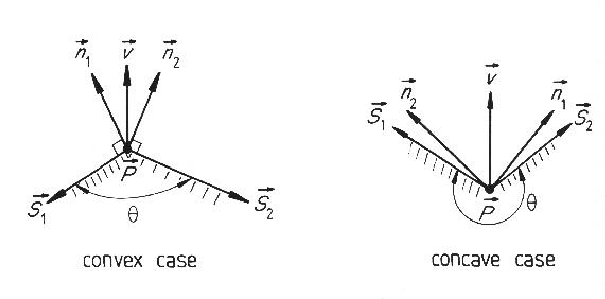Figure 6.13 shows two surfaces meeting at a boundary. Somewhere along this boundary a nominal point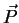is chosen and also shown is the vector of the boundary direction at that point (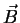). Through this point a cross-section plane is placed, such that the normals (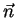) for the two surfaces lie in the plane. Figure 6.14 shows the two cases for this cross-section.

The essential information that determines the surface angle is the angle at which the two normals meet. However, it must also be determined whether the surface junction is convex or concave, which is the difficult portion of the computation. The details of the solution are seen in Figure 6.14. Let vectors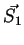and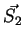be tangential to the respective surfaces in the cross-section plane. By definition, the vectoris normal to the plane in which the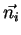and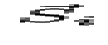vectors lie. Hence, each individualvector is normal to both the correspondingvector and thevector, and can be calculated by a cross product.

Thesevectors may face the wrong direction (e.g. away from the surface). To obtain the correct direction, a track is made from the pointin the direction of bothand. One of these should immediately enter the surface region, and this is assumed to be the correctvector.

Because the boundary must be visible, the angle between the vector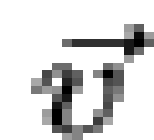from the nominal point to the viewer and a surface vectormust be less than. Hence, the angle between these vectors is guaranteed to represent open space. Then, the angle between the two surfaces is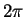minus these two open spaces. This computation is summarized below:

 Let:be a nominal point on the boundary between the two surfaces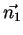,be the two surface normal vectors atbe the vector from the nominal point to the viewer Then, the boundary vectoris: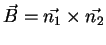and the surface vectorsare: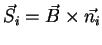which are then adjusted for direction, as described above.

Given this, the surface angle is: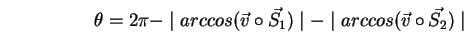The true and estimated surface angles for the modeled objects are summarized in Table 6.10. Further, only rigid angles between surfaces in the same primitive surface clusters are reported (these being the only evidence used).

Table 6.10: Summary of Relative Surface Orientation
IMAGE REGIONS ESTIMATED ANGLE TRUE ANGLE ERROR NOTE
16,26 1.47 1.57 0.10
16,29 2.96 3.14 0.18
12,18 1.53 1.57 0.04
12,31 1.60 1.57 0.03
18,31 2.03 2.14 0.11
17,25 2.09 3.14 1.05 *
17,22 1.56 1.57 0.01
* - large error across a curvature discontinuity

The estimation procedure is accurate for orientation discontinuities. The major source of errors for this process is the measurement of the surface orientation vectors by hand, and interpolating their value to the nominal point. This contributed substantially to the error at the curvature discontinuity, where interpolation flattened out the surface.Next: Relative Surface Area Up: Description of Three Dimensional Previous: Surface Elongation
Bob Fisher 2004-02-26